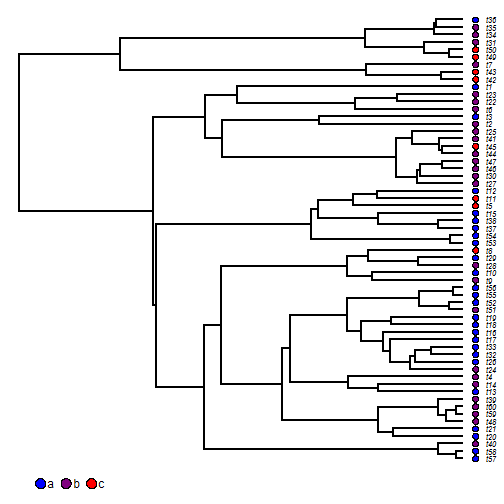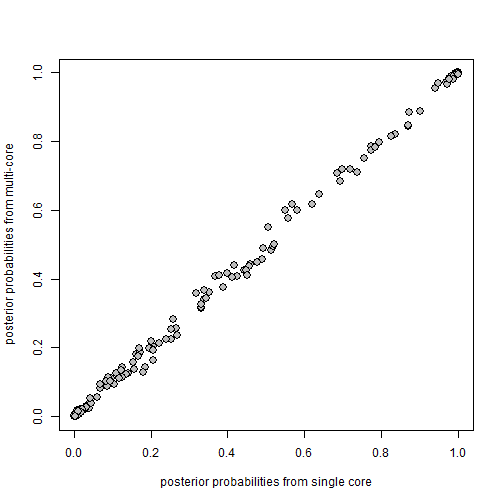## Tuesday, November 28, 2017

### Running `make.simmap` in parallel in R for Windows using snow

Shortly after I posted earlier today about parallelizing `make.simmap` using `mcapply`, which does not work in R for Windows, a colleague commented that this could indeed be done in Windows, just with slightly greater difficulty.

In fact, this turns out to be precisely true using the CRAN package snow.

Below I demonstrate that this works & that it results (on my machine, which has two cores but 4 hyper-threaded logical processors) in about a 50% speed-up relative to running the same process on a single core.

First, here's our data again:

``````library(phytools)
cols<-setNames(colorRampPalette(c("blue","red"))(3),
c("a","b","c"))
dotTree(tree,x,fsize=0.7,ftype="i",colors=cols)
``````Next we'll fit our model so we don't have to recompute the transition matrix Q for each process.

``````fit<-fitMk(tree,x,model="ARD")
fittedQ<-matrix(NA,length(fit\$states),length(fit\$states))
fittedQ[]<-c(0,fit\$rates)[fit\$index.matrix+1]
diag(fittedQ)<-0
diag(fittedQ)<--rowSums(fittedQ)
colnames(fittedQ)<-rownames(fittedQ)<-fit\$states
``````

Now let's run our analysis, first on a single core:

``````system.time(trees.sc<-make.simmap(tree=tree,x=x,Q=fittedQ,nsim=1000))
``````
``````## make.simmap is sampling character histories conditioned on the transition matrix
##
## Q =
##            a           b          c
## a -0.6678208  0.66782077  0.0000000
## b  0.3374750 -0.71533391  0.3778589
## c  0.9894179  0.07069738 -1.0601152
## (specified by the user);
## and (mean) root node prior probabilities
## pi =
##         a         b         c
## 0.3333333 0.3333333 0.3333333
``````
``````## Done.
``````
``````##    user  system elapsed
##   47.60    0.06   47.90
``````
``````trees.sc
``````
``````## 1000 phylogenetic trees with mapped discrete characters
``````

now on multiple cores using snow:

``````library(snow)
cl<-makeSOCKcluster(rep("localhost",4))
system.time(trees.mc<-clusterApply(cl,x=replicate(4,x,simplify=FALSE),
fun=make.simmap,tree=tree,Q=fittedQ,nsim=250))
``````
``````##    user  system elapsed
##    0.11    0.07   24.94
``````
``````trees.mc<-do.call(c,trees.mc)
if(!("multiSimmap"%in%class(trees.mc)))
class(trees.mc)<-c("multiSimmap",class(trees.mc))
stopCluster(cl)
trees.mc
``````
``````## 1000 phylogenetic trees with mapped discrete characters
``````

I did one peculiar thing which is sending a list of length equivalent to the number of cores consisting of replicates of our character vector `x`. This is because both `clusterApply` and `make.simmap` take the argument `x`. I could have instead written a simple wrapper function with different argument names if I wanted.

It's easy to show that our results are (more or less - remember this is stochastic mapping) identical in the two cases:

``````plot(summary(trees.sc)\$ace,summary(trees.mc)\$ace,cex=1.5,pch=21,
bg="grey",xlab="posterior probabilities from single core",
ylab="posterior probabilities from multi-core")
``````That's pretty neat right?

Thanks to Will Gearty for the tip.